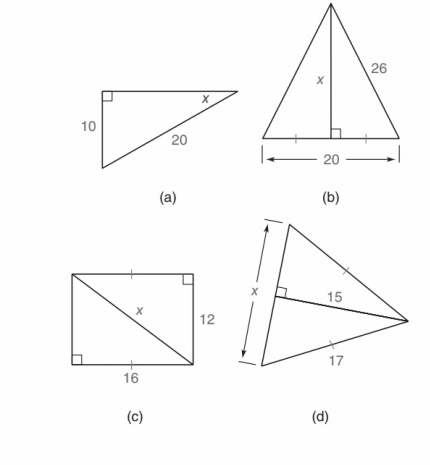Chapter 5.CR, Problem 32CRElementary Geometry For College St...

7th Edition
Alexander + 2 others
ISBN: 9781337614085

Solutions

Chapter
SectionElementary Geometry For College St...

7th Edition
Alexander + 2 others
ISBN: 9781337614085
Textbook Problem

In drawings shown, find x.To determine

a)

To find:

x

Explanation

Given:

Approach:

By using sinθ formula.

That is,

sinθ=oppositehypotenuse

Let x be the angle, opposite side is 10 and hypotenuse side is 20.

Then we have,

sinx=1020

To determine

b)

To find:

The value of x.

To determine

c)

To find:

The value of x.

To determine

d)

To find:

The value of x.

Still sussing out bartleby?

Check out a sample textbook solution.

See a sample solution

The Solution to Your Study Problems

Bartleby provides explanations to thousands of textbook problems written by our experts, many with advanced degrees!

Get Started

1340 Calculate y. y=tan1x

Calculus (MindTap Course List)

In Exercises 2340, find the indicated limit. 40. limx3xx2+72x2x+3

Applied Calculus for the Managerial, Life, and Social Sciences: A Brief Approach

In Exercises 1-6, simplify the expression. 11(1x)

Calculus: An Applied Approach (MindTap Course List)

Simplify: 180216

Elementary Technical Mathematics

Prove that limx0x2cos(1/x2)=0.

Single Variable Calculus: Early Transcendentals, Volume I

In Exercises 516, evaluate the given quantity. log218

Finite Mathematics and Applied Calculus (MindTap Course List)

If f(x) 5 for all x [2, 6] then 26f(x)dx. (Choose the best answer.) a) 4 b) 5 c) 20 d) 30

Study Guide for Stewart's Single Variable Calculus: Early Transcendentals, 8th